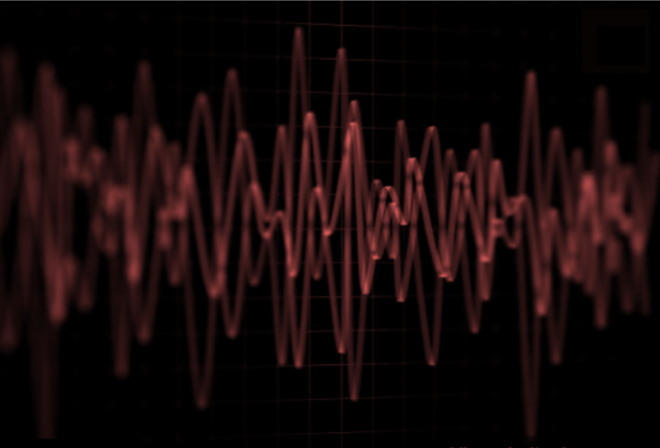# Lab Talk# Non Sinusoidal and Non Stationary Properties of Neural Signals

Electrical signals from the brain are not simply a superposition of sinusoids resulting in limitations of fourier analysis.  A recently proposed cycle-by-cycle approach can shed light on non stationary and aperiodic aspects of the signal.

In the previous blogpost we saw the issues with applying Fourier analysis to nonsinusoidal neural oscillations. Recently Cole et al  have proposed a new approach to characterize nonsinusoidal aspects of neural signals that extends the application of fourier analysis.

Computing features in time-domainThe approach begins by taking a raw neural signal (shown in 1 of the Fig to the left adapted from Cole et al, 2018), filtering it into a frequency band of interest (shown in 2 on the left for theta band filtering) and computing certain features for each cycle (which starts and ends at consecutive peaks).

First, Peaks and troughs are identified in relation to the zero-crossing point of the signal (marked in 3 in the Fig to the left). That is, a peak is defined as the maxima between two zero-crossing points (when the signal is above zero) and consequently, a trough is defined as the minima between two zero-crossing points (when the signal falls below zero). Then time-points at which the voltage is halfway between successive peak and troughs are identified as flank midpoints.After defining these points of a given signal, Cole et al propose different measures that give an insight into the shape of a waveform . For example, they define peak-trough symmetry  as follows. Let time  between two zero-crossings which contain a peak be represented as P and the time between two zero-crossings which contain a trough be represented as T. Then the peak-trough symmetry is simply given as  P / (P + T). Similarly they define rise-decay symmetry as follows. Let  time taken to reach a trough point from zero-crossing be given as D and time taken to reach a peak from the same zero-crossing be R. The rise-decay symmetry is given as R/(R + D).  For a pure sinusoidal signal, these values for a cycle will be 0.5! So these measures can also give an indication of how much the shape deviates from a sine wave.

One could similarly devise other measures of symmetry such as the ratio of the peak and trough values (the vertical distance from baseline to peak/ (vertical distance from baseline to peak + vertical distance from baseline to trough) which would give you a view of the amplitude symmetry within a cycle.

### What qualifies as an oscillation in a neural signal?Such cycle-by-cycle analysis can be used to identify whether any particular period of the segment is sinusoidal. A cycle has to satisfy three requirements to be considered sinusoidal – 1) amplitude consistency, 2) period consistency and 3) monotonicity of rise and decay periods. These are illustrated pictorially below. In Figure (A) below, both the period and amplitude are consistent for each cycle and as well as the flanks are monotonic. In Figure (B), the amplitude is inconsistent whereas in Figure (C), the period is inconsistent. In case of Figure (D), the decay flank of the second cycle is not monotonic whereas in E all the three conditions are not met. Additionally (F) has too few cycles.  Thus by plotting these symmetry metrics over time for the signal one could, in theory, potential observe oscillatory periods vs more asymmetric ones.  In the EEG for instance, alpha oscillations appear visible in short bursts which may be easily identified using such an approach.

### An example of distinct neural signalsBy computing these two measures (peak-trough symmetry) and (rise-decay symmetry) for each cycle of a neural signal, one can also look at their distributions. These distributions could be a neat way to compare neural signals with different oscillation properties. As an example, Cole et al compare recordings from CA1 and EC3 in a rat (shown in black and red), obtained from the jupyter notebook related to  provided here .

Here you can see that the peak-trough symmetry and rise-decay symmetry for these signals after applying a bandpass of (1-25 Hz) to remove slow transients.  Each have different distributions suggesting that although they have a similar spectral profile and look similar to the eye they have different characteristics.

### Implications of cycle symmetry features

There are various interesting aspects to these measures of cycle symmetry.

First, the position of the peak of the symmetry distributions (to the left or right of the 0.5 line) provides an insight into the shape of the ‘oscillation’ and how much it deviates from a sinusoid.

Second it is well known that a Fourier based approach does not handle non-stationarity well (signals whose statistical properties such as mean, variance and autocorrelation change over time).  The change in these symmetry metrics over time could identify bursts of oscillations or the degree of nonstationarity in the signal.

Third, measures such as the standard deviation, variance and skewness of the distributions of these symmetry measures provide a view into the degree of aperiodicity.  The narrower the distribution the more characteristic/similar the cycles are.  Conversely the broader the distribution the more complex and nonperiodic in nature the signal is.

### In Conclusion

The cycle-by-cycle approach provides a view into the nonsinusoidal and non stationary aspects of the signal, extending insights beyond Fourier-based analysis.  However, it has to be noted, as mentioned by the authors themselves, that the analysis itself relies on narrowband filtering to localize oscillations (for instance theta oscillations), or usage of bandpass filters to remove slow transients/high frequencies.  Thus how filters are selected will have huge influence on the result. Another challenge could be the selection of appropriate parameters to set the thresholds for defining oscillatory bursts. Nonetheless, this new approach can be seen as a complementary extension to Fourier analysis.

### References

1] Cole, Scott R., and Bradley Voytek. “Cycle-by-cycle analysis of neural oscillations.” bioRxiv (2018): 302000.

2]  Figures generated from : https://github.com/voytekresearch/Cole_2018_cyclebycycle/